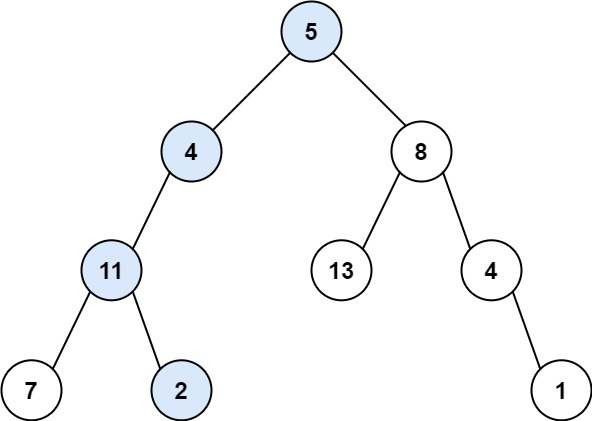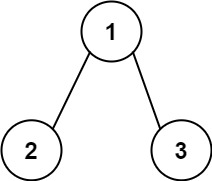# 112. Path Sum

Easy
Given the `root` of a binary tree and an integer `targetSum`, return `true` if the tree has a root-to-leaf path such that adding up all the values along the path equals `targetSum`.
A leaf is a node with no children.
Example 1:Input: root = [5,4,8,11,null,13,4,7,2,null,null,null,1], targetSum = 22
Output:
true
Explanation:
The root-to-leaf path with the target sum is shown.
Example 2:Input: root = [1,2,3], targetSum = 5
Output:
false
Explanation:
There two root-to-leaf paths in the tree:
(1 --> 2): The sum is 3.
(1 --> 3): The sum is 4.
There is no root-to-leaf path with sum = 5.
Example 3:
Input: root = [], targetSum = 0
Output:
false
Explanation:
Since the tree is empty, there are no root-to-leaf paths.
Constraints:
• The number of nodes in the tree is in the range `[0, 5000]`.
• `-1000 <= Node.val <= 1000`
• `-1000 <= targetSum <= 1000`

### 解題

/**
* Definition for a binary tree node.
* type TreeNode struct {
* Val int
* Left *TreeNode
* Right *TreeNode
* }
*/
func hasPathSum(root *TreeNode, targetSum int) bool {
if root==nil {
return false
}
if targetSum-root.Val==0 && root.Left==nil && root.Right==nil {
return true
}
if root.Left==nil {
return hasPathSum(root.Right, targetSum-root.Val)
}
if root.Right==nil {
return hasPathSum(root.Left, targetSum-root.Val)
}
leftSide := hasPathSum(root.Left, targetSum-root.Val)
rightSide := hasPathSum(root.Right, targetSum-root.Val)
if leftSide||rightSide {
return true
}
return false
}

func hasPathSum(root *TreeNode, targetSum int) bool {
if root==nil { return false }
if root.Left==nil && root.Right==nil { return root.Val == targetSum }
return hasPathSum(root.Left, targetSum-root.Val) || hasPathSum(root.Right, targetSum-root.Val)
}